# RD Sharma Solutions Class 8 Understanding Shapes Quadrilaterals Exercise 16.1

## RD Sharma Solutions Class 8 Chapter 16 Exercise 16.1

Exercise 16.1

Q 1. Define the following terms:

SOLUTION:

(i) A quadrilateral is a polygon having 4 sides (or edges) and 4 vertices (or corners). So, we can say that any closed shape having four sides is a quadrilateral.

(ii) If a mathematical figure has all internal angles equal to or less than 180 degrees, then it is known as a convex quadrilateral.

Q 2. In a quadrilateral, define each of the following:

(i) Sides

(ii) Vertices

(iii) Angles

(iv) Diagonals

(vii) Opposite sides

(viii) Opposite angles

(ix) Interior

(x) Exterior

SOLUTION:

(i) In a quadrilateral PQRS, the 4 line segments PQ, QR, RS and SP are called its sides.

(ii) The four corners of a quadrilateral are called the four vertices.

(iii)An angle is the meeting point of 2 sides of a quadrilateral and so a quadrilateral has 4 angles.

(iv) In a quadrilateral PQRS, the line segments PR and QS will be its diagonals.

(v) The angles having their arm as a common side is called adjacent angles.

(vi) if 2 sides have a common endpoint then they are adjacent.

(vii)  if 2 sides are opposite, they do not have a common endpoint.

(viii) The two non-adjacent angles of a quadrilateral are called opposite angles.

(ix) Interior is that part of the plane of a quadrilateral which encloses all the points in it.

(x) Exterior is that part of the plane of a quadrilateral which does not encloses all the points in it.

Q 3. Complete each of the following, so as to make a true statement:

(i) A quadrilateral has ….. sides.

(ii) A quadrilateral has ……. angles.

(iii) A quadrilateral has …….. vertices, no three of which are ……..

(iv) A quadrilateral has …. diagonals.

(v) The number of pairs of adjacent angles of a quadrilateral is ………

(vi) The number of pairs of opposite angles of a quadrilateral is …….

(vii) The sum of the angles of a quadrilateral is ………

(viii) A diagonal of a quadrilateral is a line segment that joins two ……… vertices of the quadrilateral.

(ix) The sum of the angles of a quadrilateral is …. right angles.

(x) The measure of each angle of a convex quadrilateral is ….. 180°.

(xi) In a quadrilateral, the point of intersection of the diagonals lies in …… of the quadrilateral.

(xii) A point is in the interior of a convex quadrilateral if it is in the ….. of its two opposite angles.

(xiii) A quadrilateral is convex if for each side, the remaining …. lie on the same side of the line containing the side.

SOLUTION:

(i) four

(ii) four

(iii) four, collinear

(iv) two

(v) four

(vi) two

(vii) 360°

(viii) opposite

(ix) four

(x) less than

(xi) the interior

(xii) interiors

(xiii) vertices

Q 4. In Fig. 16.19, ABCD is a quadrilateral.

(i) Name a pair of adjacent sides.

(ii) Name a pair of opposite sides.

(iii) How many pairs of adjacent sides are there?

(iv) How many pairs of opposite sides are there?

(v) Name a pair of adjacent angles.

(vi) Name a pair of opposite angles.

(vii) How many pairs of adjacent angles are there?

(viii) How many pairs of opposite angles are there?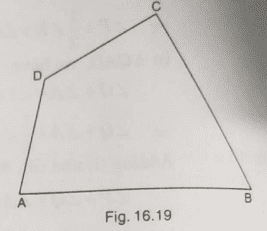SOLUTION:

(i) (AB, BC) or (BC, CD) or (CD, DA) or (AD, AB)

(ii) (AB, CD) or (BC, DA)

(iii) Four

(iv) Two

(v) ($\angle$A, $\angle$B) or ($\angle$B, $\angle$C) or ($\angle$C, $\angle$D) or ($\angle$D, $\angle$A)

(vi) ($\angle$A, $\angle$C) or ($\angle$B, $\angle$D)

(vii) Four

(viii) Two

Q 5. The angles of a quadrilateral are 110°, 72°, 55° and x°. Find the value of x.

SOLUTION:

The sum of the four angles of a quadrilateral is 360°.

Hence, we get 110° + 72° + 55° + x = 360°

237° + x = 360°

x = 360° – 237°

Thus, x = 123°

Q 6. The three angles of a quadrilateral are respectively equal to 110°, 50° and 40°. Find its fourth angle.

SOLUTION:

Let us assume y be the 4th angle.

As we know, the sum of all the 4 angles of a quadrilateral is 360°, we will have :

110° + 50° + 40° + y = 360°

200° + y = 360 °

y = 160°

Thus, The 4th angle is 160°.

Q 7. A quadrilateral has three acute angles each measure 80°. What is the measure of the fourth angle?

SOLUTION:

Let us assume y be the 4th angle.

As the sum of all 4 angles of a quadrilateral is 360°, we will have :

80° + 80° + 80° + y = 360°

240° + y = 360°

y = 120°

Thus, The 4th angle is 120°.

Q 8. A quadrilateral has all its four angles of the same measure. What is the measure of each?

SOLUTION:

Let us assume y be the measure of each angle.

As the sum of all the 4 angles of a quadrilateral is 360°, we will  have :

y° + y° + y° + y° = 360°

4y° = 360°

y° = 90°

Thus, the measure of each angle of the quadrilateral is 90°.

Q 9. Two angles of a quadrilateral are of measure 65° and the other two angles are equal. What is the measure of each of these two angles?

SOLUTION:

Let us assume y be the measure of each angle.

As the sum of all the 4  angles of a quadrilateral is 360°, we will have :

65° + 65° + y° + y° = 360°

2y° + 130° = 360°

2y° = 230°

y° = 115°

Thus, the measure of each angle of the quadrilateral is 115°.

Q 10. Three angles of a quadrilateral are equal. The fourth angle is of measure 150°. What is the measure of equal angles?

SOLUTION:

Let us assume y  be the measure of all the equal angles of the quadrilateral.

As, the sum of all the 4 angles of a quadrilateral is 360°, we will have :

y° + y° + y° + 150° = 360°

3y° = 360° – 150°

y° = 210°

Thus, the measure of each angle of the quadrilateral is 70°.

Q 11. The four angles of a quadrilateral are as 3 : 5 : 7 : 9. Find the angles.

SOLUTION:

Let all the angles of the quadrilateral be in the ratio 3y : 5y: 7y : 9y.

As the sum of all the 4 angles of a quadrilateral is 360°, we will have :

3y + 5y + 7y + 9y = 360°

24y = 360°

y = 15°

Therefore, the angles are :

3y = 45°

5y = 75°

7y = 105°

9y = 135°

Q 12. If the sum of the two angles of a quadrilateral is 180°. What is the sum of the remaining two angles?

SOLUTION:

Let us assume (y + z) be the sum of the remaining 2 angles.

As the sum of all the 4 angles of a quadrilateral is 360°, we will  have :

180° + (y + z) ° = 360°

(y + z) ° = 180°

Thus, the sum of the remaining 2 angles is 180°.

Q 13. In Fig. 16.20, find the measure of $\angle$MPN.SOLUTION:

As the sum of all the 4 angles of a quadrilateral is 360°, we will have :

45° + 90° + 90° + $\angle$MPN = 360°

225° + $\angle$MPN = 360°

Thus, $\angle$MPN = 135°

Q 14. The sides of a quadrilateral are produced in order. What is the sum of the four exterior angles?

SOLUTION:

The sum of the interior angles of quadrilateral + sum of exterior angles of the quadrilateral = 180° $\times$ 4 = 720°

As the sum of the 4 interior angles of a quadrilateral is 360°, we will have :

w + x + y + z = 360°

Putting the value, we will get :

a + b + c + d = 360°

Sum of the 4 exterior angles = 360 °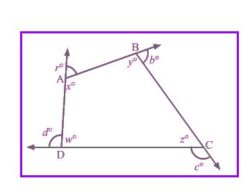Q 15. In Fig.16.21, the bisectors of $\angle$A and $\angle$B meet at a point P. If $\angle$C =100° and $\angle$D = 50°, find the measure of $\angle$APB.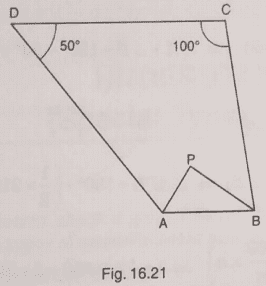SOLUTION:

$\angle$A + $\angle$B + $\angle$C + $\angle$D = 360°

$\angle$A + $\angle$B + 100° + 50° = 360°

$\angle$A + $\angle$B = 210° … (a)

In the triangle APB, we have :

$\frac{1}{2}$ $\angle$A + $\frac{1}{2}$ $\angle$B + $\angle$APB = 180°

$\angle$APB = 180° – $\frac{1}{2}$ ($\angle$A + $\angle$B)

From (a), we will get :

$\angle$APB = 180° – ($\frac{1}{2}$ $\times$210°)

Thus, $\angle$APB = 75°

Q 16. In a quadrilateral ABCD, the angles A, B, C and D are in the ratio 1 : 2 : 4 : 5. Find the measure of each angle of the quadrilateral.

SOLUTION:

Let us assume y  be the measure of each angle.

Then the ratio will become y : 2y : 4y : 5y.

Thus, the sum of all 4 angles in a quadrilateral is 360°, we will have :

y + 2y + 4y + 5y = 360°

12y = 360°

y = $\frac{360^{\circ}}{12^{\circ}}$

y = 30°

Therefore, the angles are :

y = 30°

2y = 60°

4y = 120°

5y = 150°

Q 17. In a quadrilateral ABCD, CO and DO are the bisectors of $\angle$C and $\angle$D respectively. Prove that $\angle$COD = —1 ($\angle$A + $\angle$B).

SOLUTION: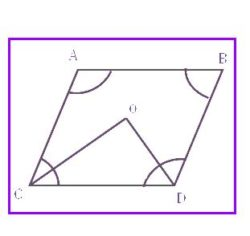$\angle$COD = 180° – ($\angle$OCD + $\angle$ODC)

= 180° – $\frac{1}{2}$ ($\angle$C + $\angle$D)

= 180° – $\frac{1}{2}$ [360° – ($\angle$A + $\angle$B)]

= 180° – 180° + $\frac{1}{2}$ ($\angle$A + $\angle$B)

= $\frac{1}{2}$ ($\angle$A + $\angle$B)

= RHS

Hence it is proved.

Q 18. Find the number of sides of a regular polygon, when each of its angles has a measure of

(i) 160° (ii) 135° (iii) 175° (iv) 162° (v) 150°

SOLUTION:

(i) Each interior angle of polygon = $\left ( \frac{2n-4}{n}\times 90 \right )^{\circ}$

So, $\left ( \frac{2n-4}{n}\times 90 \right )^{\circ}$ = 160°

$\Rightarrow \frac{2n-4}{n}=\frac{160^{\circ}}{90^{\circ}}$

$\Rightarrow \frac{2n-4}{n}=\frac{16}{9}$

$\Rightarrow 18n-36=16n$

$\Rightarrow 2n=36$

Thus, n = 18

(ii) Each interior angle  of polygon = $\left ( \frac{2n-4}{n}\times 90 \right )^{\circ}$

So, $\left ( \frac{2n-4}{n}\times 90 \right )^{\circ}$ = 135°

$\Rightarrow \frac{2n-4}{n}=\frac{135^{\circ}}{90^{\circ}}$

$\Rightarrow \frac{2n-4}{n}=\frac{3}{2}$

$\Rightarrow 4n-8=3n$

$\Rightarrow n=8$

Thus, n = 8

(iii) Each interior angle  of polygon = $\left ( \frac{2n-4}{n}\times 90 \right )^{\circ}$

Thus, $\left ( \frac{2n-4}{n}\times 90 \right )^{\circ}$ = 175°

$\Rightarrow \frac{2n-4}{n}=\frac{175^{\circ}}{90^{\circ}}$

$\Rightarrow \frac{2n-4}{n}=\frac{35}{18}$

$\Rightarrow 36n-72=35n$

$\Rightarrow n=72$

therefore, n = 72

(iv) Each interior angle of polygon = $\left ( \frac{2n-4}{n}\times 90 \right )^{\circ}$

Therefore, $\left ( \frac{2n-4}{n}\times 90 \right )^{\circ}$ = 162°

$\Rightarrow \frac{2n-4}{n}=\frac{162^{\circ}}{90^{\circ}}$

$\Rightarrow \frac{2n-4}{n}=\frac{9}{5}$

$\Rightarrow 10n-20=9n$

$\Rightarrow n=20$

Thus, n = 20

(v) Each interior angle of polygon = $\left ( \frac{2n-4}{n}\times 90 \right )^{\circ}$

Therefore, $\left ( \frac{2n-4}{n}\times 90 \right )^{\circ}$ = 150°

$\Rightarrow \frac{2n-4}{n}=\frac{150^{\circ}}{90^{\circ}}$

$\Rightarrow \frac{2n-4}{n}=\frac{5}{3}$

$\Rightarrow 6n-12=5n$

$\Rightarrow n=12$

Thus, n = 12.

Q 19. Find the number of degrees in each exterior angle of a regular pentagon.

SOLUTION:

Each exterior angle of pentagon = $\left (\frac{360}{n} \right )^{\circ}$

For a regular pentagon, n will be = 5.

Thus, exterior angle = $\left (\frac{360}{5} \right )^{\circ}$ = 72 $^{\circ}$

Q 20. The measure of angles of a hexagon are x°, (x – 5)°, (x – 5)°, (2x – 5)°, (2x – 5)°, (2x + 20)°. Find the value of x.

SOLUTION:

Therefore the sum of all the 6 angles of a hexagon is 720°, we will get :

x° + (x – 5)° + (x – 5)° + (2x – 5)° + (2x – 5)° + (2x + 20) ° = 720°

x° + x° – 5° + x° – 5° + 2x – 5° + 2x – 5° + 2x + 20° = 720°

9x – 20° + 20° = 720°

9x = 720°

Thus, x = 80

Q 21. In a convex hexagon, prove that the sum of all interior angle is equal to twice the sum of its exterior angles formed by producing the sides in the same order.

SOLUTION:

For a convex hexagon, interior angle = $\left ( \frac{2n-4}{n}\times 90 \right )^{\circ}$

For a hexagon, n = 6

Thus, Interior angle = $\left ( \frac{12-4}{6}\times 90 \right )^{\circ}$

= $\left ( \frac{8}{6}\times 90 \right )^{\circ}$

= 120°

Therefore, the sum of all the 6 interior angles = 120° + 120° + 120° + 120° + 120° + 120° = 720°

Thus, Exterior angle = $\left (\frac{360}{n} \right )^{\circ}$ = $\left (\frac{360}{6} \right )^{\circ}$ = 60°

Hence, sum of all the exterior angles = 60° + 60° + 60° + 60° + 60° + 60° = 360°

Now, sum of all interior angles = 720°

= 2(360°)

= two times the exterior angles

Hence it is proved.

Q 22. The sum of the interior angles of a polygon is three times the sum of its exterior angles. Determine the number of sides of the polygon.

SOLUTION:

{(2n – 4) $\times$ 90°} = 3 $\times$ ($\left (\frac{360}{n} \right )^{\circ}$ $\times$ n)

=> (n – 2) $\times$180 = 3 $\times$360

=> n – 2 = 6

Thus, n = 8.

Q 23. Determine the number of sides of a polygon whose exterior and interior angles are in the ratio 1 : 5.

SOLUTION:

Let us assume p be the number of sides of the polygon.

Let us assume a and 5b be the exterior and interior angles respectively.

Therefore the sum of an interior & the corresponding exterior angle is 180°, we will have :

a + 5b = 180°

6b = 180°

b = 30°

As the polygon has p sides.

Hence, the sum of all the exterior angles = (30p)°

The sum of all the exterior angles of a polygon is 360°.

i.e., 30p = 360

Thus, p = 12.

Q 24. PQRSTU is a regular hexagon. Determine each angle of triangle PQT.

SOLUTION: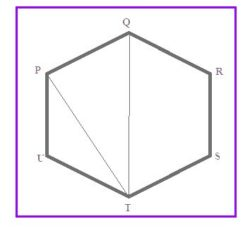As we know that regular hexagon is made up of six equilateral triangles.

Therefore, $\angle$PQT = 60° and $\angle$QTP = 30°

As the sum of the angles of trianglePQT is 180°, we will  have :

$\angle$P + $\angle$Q + $\angle$T = 180°

$\angle$P + 60° + 30° = 180°

$\angle$P = 180° – 90°

$\angle$QQPT = 90°

So, the angles of the triangle are 90°, 60° and 30°.